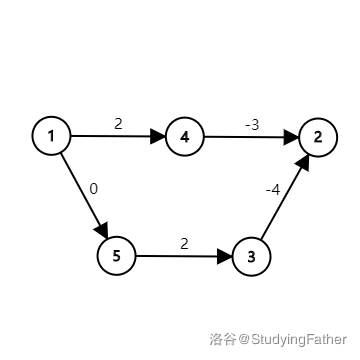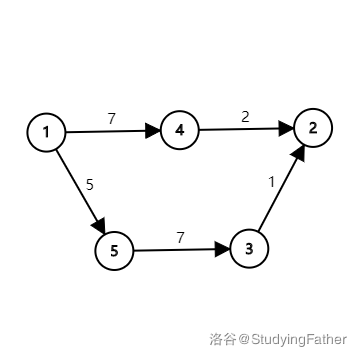# Studying Father's luogu blog

Focus on interest!

# [洛谷日报#242]Johnson 全源最短路径算法学习笔记

Johnson 和 Floyd 一样，是一种能求出无负环图上任意两点间最短路径的算法。该算法在 1977 年由 Donald B. Johnson 提出。

## 1 算法概述$1 \to 2$ 的最短路为 $1 \to 5 \to 3 \to 2$，长度为 $-2$。Johnson 算法则通过另外一种方法来给每条边重新标注边权。

Q：那这么说，Dijkstra 也可以求出负权图（无负环）的单源最短路径了？
A：没错。但是预处理要跑一遍 Bellman-Ford，还不如直接用 Bellman-Ford 呢。

## 2 正确性证明

$(w(s,p_1)+h_s-h_{p_1})+(w(p_1,p_2)+h_{p_1}-h_{p_2})+ \dots +(w(p_k,t)+h_{p_k}-h_t)$

$w(s,p_1)+w(p_1,p_2)+ \dots +w(p_k,t)+h_s-h_t$

## 3 参考代码

（被各位 D 惨了，所以把代码扔到 clang-format 里格式化了下 /wq）

#include <cstring>
#include <iostream>
#include <queue>
#define INF 1e9
using namespace std;
struct edge {
int v, w, next;
} e;
struct node {
int dis, id;
bool operator<(const node& a) const { return dis > a.dis; }
node(int d, int x) { dis = d, id = x; }
};
int cnt, n, m;
long long h, dis;
void addedge(int u, int v, int w) {
e[++cnt].v = v;
e[cnt].w = w;
}
bool spfa(int s) {
queue<int> q;
memset(h, 63, sizeof(h));
h[s] = 0, vis[s] = 1;
q.push(s);
while (!q.empty()) {
int u = q.front();
q.pop();
vis[u] = 0;
for (int i = head[u]; i; i = e[i].next) {
int v = e[i].v;
if (h[v] > h[u] + e[i].w) {
h[v] = h[u] + e[i].w;
if (!vis[v]) {
vis[v] = 1;
q.push(v);
t[v]++;
if (t[v] == n + 1) return false;
}
}
}
}
return true;
}
void dijkstra(int s) {
priority_queue<node> q;
for (int i = 1; i <= n; i++) dis[i] = INF;
memset(vis, 0, sizeof(vis));
dis[s] = 0;
q.push(node(0, s));
while (!q.empty()) {
int u = q.top().id;
q.pop();
if (vis[u]) continue;
vis[u] = 1;
for (int i = head[u]; i; i = e[i].next) {
int v = e[i].v;
if (dis[v] > dis[u] + e[i].w) {
dis[v] = dis[u] + e[i].w;
if (!vis[v]) q.push(node(dis[v], v));
}
}
}
return;
}
int main() {
ios::sync_with_stdio(false);
cin >> n >> m;
for (int i = 1; i <= m; i++) {
int u, v, w;
cin >> u >> v >> w;
}
for (int i = 1; i <= n; i++) addedge(0, i, 0);
if (!spfa(0)) {
cout << -1 << endl;
return 0;
}
/*
for(int i=1;i<=n;i++)
cout<<h[i]<<' ';
cout<<endl;
*/
for (int u = 1; u <= n; u++)
for (int i = head[u]; i; i = e[i].next) e[i].w += h[u] - h[e[i].v];
for (int i = 1; i <= n; i++) {
dijkstra(i);
long long ans = 0;
for (int j = 1; j <= n; j++) {
if (dis[j] == INF)
ans += j * INF;
else
ans += j * (dis[j] + h[j] - h[i]);
}
cout << ans << endl;
}
return 0;
}

## Reference

2019-10-28 19:15:45 in 日报